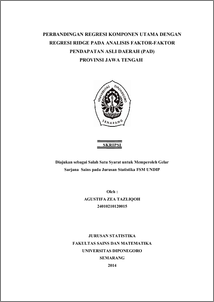# PERBANDINGAN REGRESI KOMPONEN UTAMA DENGAN REGRESI RIDGE PADA ANALISIS FAKTOR-FAKTOR PENDAPATAN ASLI DAERAH (PAD) PROVINSI JAWA TENGAH

TAZLIQOH, AGUSTIFA ZEA (2015) PERBANDINGAN REGRESI KOMPONEN UTAMA DENGAN REGRESI RIDGE PADA ANALISIS FAKTOR-FAKTOR PENDAPATAN ASLI DAERAH (PAD) PROVINSI JAWA TENGAH. Undergraduate thesis, FSM Universitas Diponegoro.Preview
PDF
4Mb

## Abstract

Assuming violation multicollinearity in classical regression analysis can cause estimator resulting from classical model regression inefficient. Principal components regression and ridge regression are the methods that can be used to overcome the problem of multicollinearity. This research aimed to compare between the principal components regression with ridge regression to tackle the problem of multicollinearity in the analysis of the factors that affect revenue (PAD) of the Central Java province. The data used in this research are data revenue (PAD), and factors that affect the region, such as local tax, retribution, Gross Regional Domestic Products (GRDP) at current prices, Gross Regional Domestic Products (GRDP at constant prices, population, regional spending. Based on the coefficient of determination value and test on individual regression coefficients, the value of variance inflation factor and correlations sufficiently high among some independent variables so we can conclude the existence of a violation of multicollinearity on analysis factors PAD. Based on standard error resulting from principal components regression and ridge regression show that principal components regression results in a standard smaller error. This shows that principal component regression is better than ridge regression in solving the problem multicollinearity on analysis of factors that affects pad province of central java. Keywords: Multicolinearity, revenue (PAD), Principal Component Regression, Ridge Regression, standard error

Item Type: Thesis (Undergraduate) H Social Sciences > HA Statistics Faculty of Science and Mathematics > Department of Statistics 46553 Mr Hasbi Yasin 05 Oct 2015 15:09 05 Oct 2015 15:09

Repository Staff Only: item control page